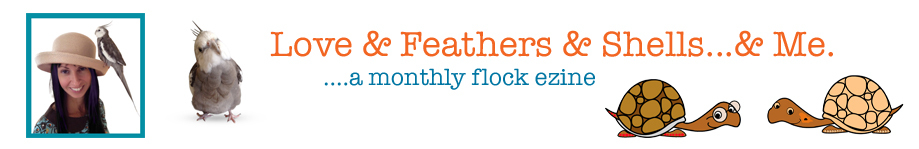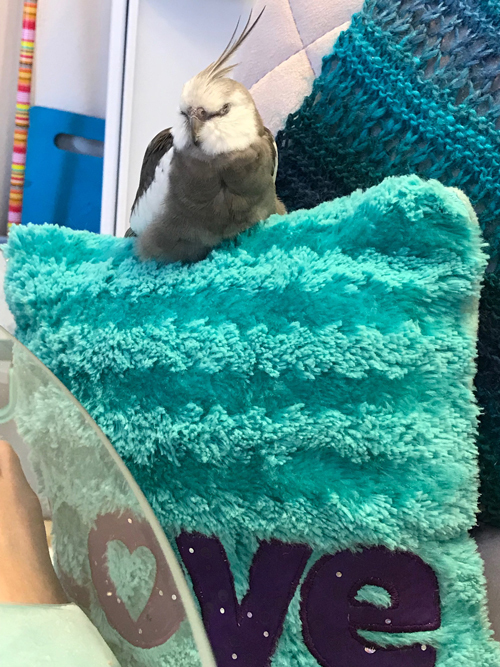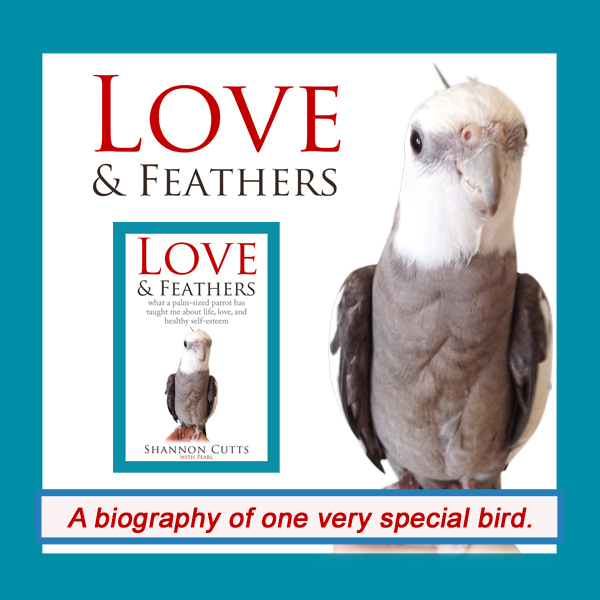# February 2018

 table div table+table+table div table{width:100%;padding:0}table div table+table+table div table img{width:96.23%;padding:0;float:none}table div table+table+table div table td{width:100%;padding:0 1.88% 18px}/* styles */## When Your Soul Feels LonelyEven feathered beings can get lonely - I know this because Pearl shrieks for me when I leave, but snoozes contentedly when I am nearby.

This world can be a lonely place for a soul.

For the body, there is so much to see and do and feel and taste and experience - and it is all right here at our fingertips. The body can easily stay so busy, and so sensorily-fulfilled, it doesn't even have time to think about whether it is lonely or not.

For the mind, whether in waking or in sleep, there is near-limitless access to stimulation and satisfying occupation. Even better, the mind and emotions are joined at the hip as they think and feel and feel and think their way through each successive second of their shared days....life-mates right down to their twin cores.

For the heart, there is the ever-present opportunity for connection. From casual group meet-ups to deeply intimate one-to-one partnerships, concrete physical to virtual encounters, the average day brings approximately 7.442 billion connection options - and that is just if it is limited to connecting with other hearts of its same species!

The soul can't find relief, release, reassurance, a replete filling-up, from any of these places. Even nature, which many mystics and sages and ordinary folks (like me) believe is as close as any sentient being can get to the formless ALL with the barrier of body-mind-emotions-heart yet standing in between, does not comfort a soul.

The soul needs something different. Or maybe it is more accurate to say it needs something deeper. Or something more trustworthy. Or something that lasts.

Rubbing two bodies together will produce a temporary pleasure. But then it wears off. A warm cup of coffee, a promotion at work, the sight of birds in flight, a fulfilling conversation, even total companionable silence between two beings - these experiences ebb and flow. They come and go.

When they go, the soul is left all alone....again.

The older I get, the more every part of my being - body, mind, emotions, heart - needs me. Each part comes to me with daily requests - for easing of pain, good health, creative stimulation, emotive expression, connection (or often, re-connection).

But my soul needs me most of all. And it doesn't even know what to ask for. And I have no idea what to offer. Everything it has tried on its own to ease the emptiness comes up short. Its ache is profound - nearly unmentionable.

Its ache is my ache.

The truth is, I need what my soul needs. I am not body, or mind, or emotions, or even heart - I am spirit. I am deeper, farther removed, in but not of, all of these things. I, too, am moving hopefully, restlessly, and increasingly exhaustedly through life, searching, seeking, asking, begging for something REAL.

I am searching for my soul. And it is searching for me.

With great respect and love,

Shannon

 table div table+table+table+table+table+table div table{width:100%;padding:0}table div table+table+table+table+table+table div table img{width:96.23%;padding:0;float:none}table div table+table+table+table+table+table div table td{width:100%;padding:0 1.88% 18px}/* styles */## What works for me to ease soul-level loneliness:

▪ Praying and meditating - just closing my eyes for a moment to reconnect within.
▪ Watching the ocean and the limitless ebb and flow of the waves.
▪ Hearing the sound of Pearl beak-grinding...a sound that tells me he feels safe and content.
▪ Sometimes writing really eases the inner loneliness because I can hear myself so clearly.
▪ Watching ladybugs - these tiny messengers show up whenever I need a sign that I am not alone.
 ▪ Praying and meditating - just closing my eyes for a moment to reconnect within.
 ▪ Watching the ocean and the limitless ebb and flow of the waves.
 ▪ Hearing the sound of Pearl beak-grinding...a sound that tells me he feels safe and content.
 ▪ Sometimes writing really eases the inner loneliness because I can hear myself so clearly.
 ▪ Watching ladybugs - these tiny messengers show up whenever I need a sign that I am not alone.
 table div table+table+table+table+table+table+table+table div table{width:100%;padding:0}table div table+table+table+table+table+table+table+table div table img{width:96.23%;padding:0;float:none}table div table+table+table+table+table+table+table+table div table td{width:100%;padding:0 1.88% 18px}/* styles */# Get your copy of Love & Feathers the book!Click on the image of Pearl to order your signed copy!Pearl will even beak-o-graph it for you!
 table div table+table+table+table+table+table+table+table+table+table+table+table+table div table{width:100%;padding:0}table div table+table+table+table+table+table+table+table+table+table+table+table+table div table img{width:96.23%;padding:0;float:none}table div table+table+table+table+table+table+table+table+table+table+table+table+table div table td{width:100%;padding:0 1.88% 18px}/* styles */# Malti is getting bigger every day!

 table.module-14{width:75.47%;padding:0}table div table+table+table+table+table+table+table+table+table+table+table+table+table+table+table div table{width:75.47%;float:none;margin-left:auto;margin-right:auto;padding:0}table div table+table+table+table+table+table+table+table+table+table+table+table+table+table+table div table a{border:0 none;text-decoration:none}table div table+table+table+table+table+table+table+table+table+table+table+table+table+table+table div table img{width:100%!important;border:0 none;text-decoration:none}table div table+table+table+table+table+table+table+table+table+table+table+table+table+table+table div table td{width:100%;padding:0}/* styles */
 table div table+table+table+table+table+table+table+table+table+table+table+table+table+table+table+table+table div table{width:100%;padding:0}table div table+table+table+table+table+table+table+table+table+table+table+table+table+table+table+table+table div table img{width:96.23%;padding:0;float:none}table div table+table+table+table+table+table+table+table+table+table+table+table+table+table+table+table+table div table td{width:100%;padding:0 1.88% 18px}/* styles */# Bruce is still in hibernation - we miss him!!

 table.module-18{width:75.47%;padding:0}table div table+table+table+table+table+table+table+table+table+table+table+table+table+table+table+table+table+table+table div table{width:75.47%;float:none;margin-left:auto;margin-right:auto;padding:0}table div table+table+table+table+table+table+table+table+table+table+table+table+table+table+table+table+table+table+table div table a{border:0 none;text-decoration:none}table div table+table+table+table+table+table+table+table+table+table+table+table+table+table+table+table+table+table+table div table img{width:100%!important;border:0 none;text-decoration:none}table div table+table+table+table+table+table+table+table+table+table+table+table+table+table+table+table+table+table+table div table td{width:100%;padding:0}/* styles */
 table div table+table+table+table+table+table+table+table+table+table+table+table+table+table+table+table+table+table+table+table+table div table{width:100%;padding:0}table div table+table+table+table+table+table+table+table+table+table+table+table+table+table+table+table+table+table+table+table+table div table img{width:96.23%;padding:0;float:none}table div table+table+table+table+table+table+table+table+table+table+table+table+table+table+table+table+table+table+table+table+table div table td{width:100%;padding:0 1.88% 18px}/* styles */## Meet Pearl, Malti, Bruce & Me

 table div table+table+table+table+table+table+table+table+table+table+table+table+table+table+table+table+table+table+table+table+table+table+table div table,table.module-22{width:34.34%;float:left;padding:0}table div table+table+table+table+table+table+table+table+table+table+table+table+table+table+table+table+table+table+table+table+table+table+table div table a{border:0 none;text-decoration:none}table div table+table+table+table+table+table+table+table+table+table+table+table+table+table+table+table+table+table+table+table+table+table+table div table img{width:100%!important;border:0 none;text-decoration:none}table div table+table+table+table+table+table+table+table+table+table+table+table+table+table+table+table+table+table+table+table+table+table+table div table td{width:100%;padding:0 20px 20px 0}/* styles */ fHi! It is so good to meet you here each month! I'm so excited about our new book, "Love & Feathers: what a palm-sized parrot has taught me about life, love, and healthy self-esteem."The book focuses on the power of animal mentors in our lives and features my parrot, Pearl. Malti is a young red-foot tortoise with a healthy appetite for food, snoozing and life! Bruce, a rescued Texas 3-toed box turtle, is our newest shelled flock member. My first book, "Beating Ana: how to outsmart your eating disorder and take your life back," shares how I recovered from an eating disorder so I could pursue my creative dreams. MentorCONNECT, the first global eating disorders nonprofit mentoring community, emerged from the experience of writing "Beating Ana." While we closed in April 2017, the website continues to provide information about mentoring-based recovery support resources. ==> To connect with me: www.shannoncutts.com ==> To connect with Pearl, Malti & Bruce: www.loveandfeathersandshells.com
 table div table+table+table+table+table+table+table+table+table+table+table+table+table+table+table+table+table+table+table+table+table+table+table+table div table{width:100%;padding:0}table div table+table+table+table+table+table+table+table+table+table+table+table+table+table+table+table+table+table+table+table+table+table+table+table div table img{width:96.23%;padding:0;float:none}table div table+table+table+table+table+table+table+table+table+table+table+table+table+table+table+table+table+table+table+table+table+table+table+table div table td{width:100%;padding:0 1.88% 18px}/* styles */table div table+table+table+table+table+table+table+table+table+table+table+table+table+table+table+table+table+table+table+table+table+table+table+table+table div table td,table.module-24{width:100%;padding:0}table div table+table+table+table+table+table+table+table+table+table+table+table+table+table+table+table+table+table+table+table+table+table+table+table+table div table{width:100%;float:none;margin-left:auto;margin-right:auto;padding:0}table div table+table+table+table+table+table+table+table+table+table+table+table+table+table+table+table+table+table+table+table+table+table+table+table+table div table a{border:0 none;text-decoration:none}table div table+table+table+table+table+table+table+table+table+table+table+table+table+table+table+table+table+table+table+table+table+table+table+table+table div table img{width:100%!important;border:0 none;text-decoration:none}/* styles */
 table.module-25{width:74.15%;padding:0}table div table+table+table+table+table+table+table+table+table+table+table+table+table+table+table+table+table+table+table+table+table+table+table+table+table+table div table{width:74.15%;float:none;margin-left:auto;margin-right:auto;padding:0}table div table+table+table+table+table+table+table+table+table+table+table+table+table+table+table+table+table+table+table+table+table+table+table+table+table+table div table a{border:0 none;text-decoration:none}table div table+table+table+table+table+table+table+table+table+table+table+table+table+table+table+table+table+table+table+table+table+table+table+table+table+table div table img{width:100%!important;border:0 none;text-decoration:none}table div table+table+table+table+table+table+table+table+table+table+table+table+table+table+table+table+table+table+table+table+table+table+table+table+table+table div table td{width:100%;padding:0}/* styles */
 table div table+table+table+table+table+table+table+table+table+table+table+table+table+table+table+table+table+table+table+table+table+table+table+table+table+table+table div table{width:100%;padding:0}table div table+table+table+table+table+table+table+table+table+table+table+table+table+table+table+table+table+table+table+table+table+table+table+table+table+table+table div table img{width:96.23%;padding:0;float:none}table div table+table+table+table+table+table+table+table+table+table+table+table+table+table+table+table+table+table+table+table+table+table+table+table+table+table+table div table td{width:100%;padding:0 1.88% 18px}/* styles */## Reprints & Reposts

REPRINTS: Would you like to use any portion of this material in your newsletter, blog or website? You may do so as long as you also include this copyright information: